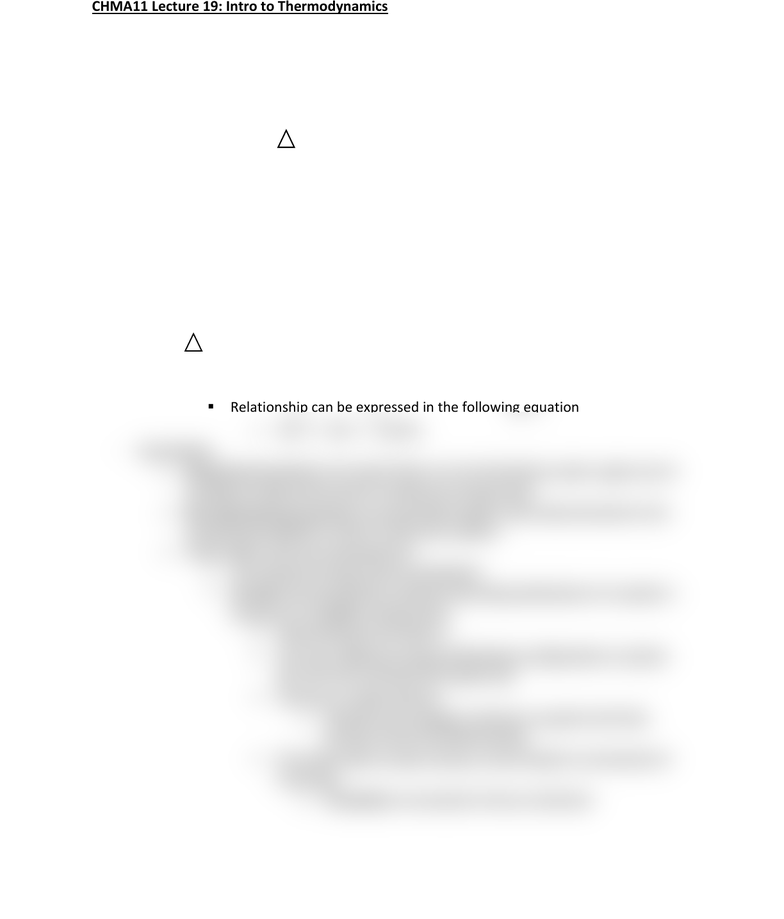# CHMA11H3 Lecture Notes - Lecture 22: Spontaneous Process, Enthalpy, Thermodynamics

62 views2 pages
School
Department
CourseCHMA11 Lecture 19: Intro to Thermodynamics
- Thermodynamics: the study of heat and its relationship with energy
o Focused on chemical relationships between heat and energy
o 1st law of thermodynamics: energy is neither created nor destroyed, it can only
be transferred from form to form
Can be expressed in the following equation
E = q + w
o q: represents heat
positive q value = heat is absorbed
negative q value = heat is released
o w: represents work
positive w value = work is done on the system
negative w value = work is done by the system
o 2nd law of thermodynamics: all spontaneous processes produce increases in the
entropy of the universe
- Enthalpy: heat involved in chemical reactions under constant pressure
o H: used to represent enthalpy
Enthalpy is equal to the change in internal energy of a system plus the
change in pressure times volume of the system
Relationship can be expressed in the following equation
H = E + (PV)
- Spontaneity
o Spontaneous process: processes that occur by themselves under a given set of
conditions without the need of continuous energy input
o Non-Spontaneous process: processes that require some external action to be
continuously applied in order to drive the reaction
o What makes a process spontaneous?
The spread of energy drives spontaneity
Entropy: thermodynamic property describing distribution of a system’s
energy over available energy levels
Represented by the letter S
The more different energy distributing configurations a system
has, the more entropy the system has
Entropy is a state function
o Therefore the change is entropy is equal to the final
entropy minus the initial entropy
Processes that increase entropy: all are based on movement of
molecules
o Translation: movement in the x/y direction
Unlock document

This preview shows half of the first page of the document.
Unlock all 2 pages and 3 million more documents.